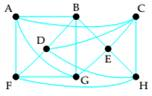# Use Dirac's theorem to verify that the graph is Hamiltonian. Then find a Hamiltonian circuit.### Mathematical Excursions (MindTap C...

4th Edition
Richard N. Aufmann + 3 others
Publisher: Cengage Learning
ISBN: 9781305965584

#### Solutions

Chapter
Section### Mathematical Excursions (MindTap C...

4th Edition
Richard N. Aufmann + 3 others
Publisher: Cengage Learning
ISBN: 9781305965584
Chapter 5.2, Problem 3ES
Textbook Problem
1 views

## Use Dirac's theorem to verify that the graph is Hamiltonian. Then find a Hamiltonian circuit.To determine

To determine if the graph is Hamiltonian using Dirac's theorem. Then to find a Hamiltonian circuit.

### Explanation of Solution

Given information:

Given, graph is:

Calculation:

Dirac's theorem states, for a connected graph with at least three vertices and no multiple edges, if the number of vertices is n and every vertex has degree of at least n/2, then the graph is a Hamiltonian graph.

Here,

The number of vertices in the graph is 8 (A, B, C, D, E, F, G, H)

So the graph is Hamiltonian if the degree of every vertex is at least 8/2=4

And,

The degree of the vertex A is 4

The degree of the vertex B is 4

The degree of the vertex C is 4

The degree of the vertex D...

### Still sussing out bartleby?

Check out a sample textbook solution.

See a sample solution

#### The Solution to Your Study Problems

Bartleby provides explanations to thousands of textbook problems written by our experts, many with advanced degrees!

Get Started

Find more solutions based on key concepts
[65,-12]

Applied Calculus for the Managerial, Life, and Social Sciences: A Brief Approach

Evaluate the integral. 33. xsecxtanxdx

Single Variable Calculus: Early Transcendentals

Reminder Round all answers to two decimal places unless otherwise indicated. Using Average Rate of Change The f...

Functions and Change: A Modeling Approach to College Algebra (MindTap Course List)

True or False: is a convergent series.

Study Guide for Stewart's Single Variable Calculus: Early Transcendentals, 8th

The third partial sum of is:

Study Guide for Stewart's Multivariable Calculus, 8th